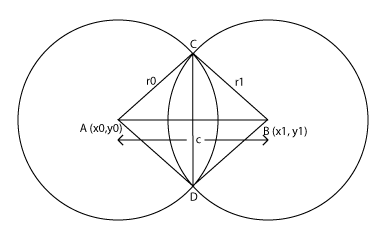# Solution Hint: Algorthimic Problem 1st (How to find the shared area of two intersected circle)

If you will go for the problem statement then you will find that you have to find the Area covered by intersection of two or three circles and the coordinates and radius is given.

Lets see how to find the shared area between intersection of two circles.Let two circles are given.

```1. center(x0,y0), radius (r0)

Let A be the centre of the circle `( x0, y0 )` and B be the centre of the other circle `( x1,y1 ).`

Draw the circles with appropriate radii r0 and r1 so that there is a reasonable amount of overlap.  The length AB is calculated from the coordinates of the centre:

`AB = sqrt{(x1-x0)^2 + (y1-y0)^2}`

For convenience let this length be denoted by c.

The two circles intersect in two points which I will label C and D.

Now we must calculate the angles CAD and CBD, and we do this using the cosine formula. In fact it is half of these angles that we first calculate, using triangle CAB.

`r0^2 = r1^2 + c^2 - 2*r1*c*cos(CBA)`
`cos(CBA) = (r1^2 + c^2 - r0^2)/(2*r1*c)`

found CBA, then CBD = 2(CBA).

Similarly,
`cos(CAB) = (r0^2 + c^2 - r1^2)/(2*r0*c)`
and then
`CAD = 2(CAB)`

Express CBD and CAD in radian measure. Then we find the segment of each of the circles cut off by the chord CD, by taking the area of the sector of the circle BCD and subtracting the area of triangle BCD.
Similarly we find the area of the sector ACD and subtract the area of triangle ACD.

```Area = ( 1/2 )( CBD ) r1 ^ 2 - ( 1/2 ) r1 ^ 2 * sin( CBD )
+ ( 1/2 )( CAD ) r0 ^ 2 - ( 1/2 ) r0 ^ 2 * sin( CAD )```

Remember that for the area of the sectors you must have CBD and CAD in radians.

One more thing if the two circles are of the SAME radius please note that the area is symmetrical about the chord CD. Therefore, you only need to find the area in one half of the intersection and multiply by 2.

A shorter equation is

` Area = 2 * ( ( 1/2 ) ( CBD ) r1 ^ 2 - ( 1/2 ) r1 ^ 2.sin( CBD ) ). `

One more derivation if the redis is equal then you can find out using, this formula
`Area = r^2*(q - sin(q))  where q = 2*acos(c/2r),`
where c = distance between centers and r is the common radius.

I think now you can solve this. If something goes wrong, then post in the comments.

### Surya

Living in permanent beta mode: Learning, Improving & evolving. SPECIALTIES: Web Application Development, Digital Media, E-Commerce Solutions, SEO, CRM Solutions, Open Source Technologies, System Administration ( Linux ), VOIP Solutions, Cloud Computing, Web Security.#### Why to buy Pixel 2 by Google? not iPhone X !#### How to set or change default text editor for crontab in Linux?### 1 comments On Solution Hint: Algorthimic Problem 1st (How to find the shared area of two intersected circle)

This site uses Akismet to reduce spam. Learn how your comment data is processed.